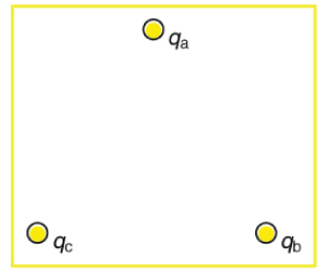# Problem: In the figure, the point charges are located at the corners of an equilateral triangle 22 cm on a side.A.) Find the magnitude of the electric field in N/C at the location of qa given that qb = 11.1 μC and qc = -5.3 μCB.) Find the direction of the electric field at qa in degrees above the negative x-axis with origin at qa.

###### FREE Expert Solution
85% (239 ratings)View Complete Written Solution
###### Problem Details

In the figure, the point charges are located at the corners of an equilateral triangle 22 cm on a side.

A.) Find the magnitude of the electric field in N/C at the location of qa given that qb = 11.1 μC and qc = -5.3 μC

B.) Find the direction of the electric field at qa in degrees above the negative x-axis with origin at qa.